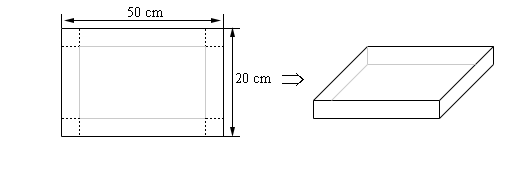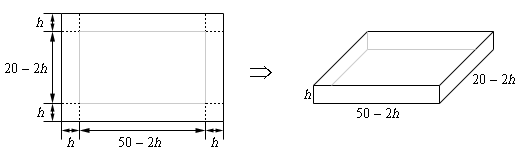Paul's Online Notes
Home / Calculus I / Applications of Derivatives / Optimization
Show Mobile Notice Show All Notes Hide All Notes
Mobile Notice
You appear to be on a device with a "narrow" screen width (i.e. you are probably on a mobile phone). Due to the nature of the mathematics on this site it is best views in landscape mode. If your device is not in landscape mode many of the equations will run off the side of your device (should be able to scroll to see them) and some of the menu items will be cut off due to the narrow screen width.

### Section 4.8 : Optimization

8. We have a piece of cardboard that is 50 cm by 20 cm and we are going to cut out the corners and fold up the sides to form a box. Determine the height of the box that will give a maximum volume.Show All Steps Hide All Steps

Start Solution

The first step is to do a quick sketch of the problem.Show Step 2

As with the problem like this in the notes the constraint is really the size of the box and that has been taken into account in the figure so all we need to do is set up the volume equation that we want to maximize.

$V\left( h \right) = h\left( {50 - 2h} \right)\left( {20 - 2h} \right) = 4{h^3} - 140{h^2} + 1000h$ Show Step 3

Finding the critical point(s) for this shouldn’t be too difficult at this point so here is that work,

$V'\left( h \right) = 12{h^2} - 280h + 1000\hspace{0.75in}h = \frac{35 \pm 5\sqrt {19}}{3} = 4.4018,\,\,18.9315$

From the figure above, we can see that the limits on $$h$$ must be $$h = 0$$ and $$h = 10$$ (the largest $$h$$ could be is ½ the smaller side). Note that neither of these really make physical sense but they do provide limits on $$h$$.

So, we must have $$0 \le h \le 10$$ and this eliminates the second critical point and so the only critical point we need to worry about is $$h = 4.4018$$

Show Step 4

Because we have limits on $$h$$ we can quickly check to see if we have maximum by plugging in the volume function.

$V\left( 0 \right) = 0\hspace{0.75in}V\left( {4.4018} \right) = 2030.34\hspace{0.75in}V\left( {10} \right) = 0$

So, we can see then that the height of the box will have to be $$\require{bbox} \bbox[2pt,border:1px solid black]{{h = 4.4018}}$$ in order to get a maximum volume.